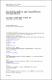## One-Way Functions Using Algorithmic and Classical Information Theories2013
##### Authors
Luís Filipe Antunes
Matos,A
Alexandre Miranda Pinto
Souto,A
Andreia Sofia Teixeira
##### Abstract
We prove several results relating injective one-way functions, time-bounded conditional Kolmogorov complexity, and time-bounded conditional entropy. First we establish a connection between injective, strong and weak one-way functions and the expected value of the polynomial time-bounded Kolmogorov complexity, denoted here by E(K-t (x vertical bar f (x))). These results are in both directions. More precisely, conditions on E(K-t (x vertical bar f (x))) that imply that f is a weak one-way function, and properties of E(K-t (x vertical bar f (x))) that are implied by the fact that f is a strong one-way function. In particular, we prove a separation result: based on the concept of time-bounded Kolmogorov complexity, we find an interval in which every function f is a necessarily weak but not a strong one-way function. Then we propose an individual approach to injective one-way functions based on Kolmogorov complexity, defining Kolmogorov one-way functions and prove some relationships between the new proposal and the classical definition of one-way functions, showing that a Kolmogorov one-way function is also a deterministic one-way function. A relationship between Kolmogorov one-way functions and the conjecture of polynomial time symmetry of information is also proved. Finally, we relate E(K-t (x vertical bar f (x))) and two forms of time-bounded entropy, the unpredictable entropy H-unp, in which "one-wayness" of a function can be easily expressed, and the Yao(+) entropy, a measure based on compression/decompression schema in which only the decompressor is restricted to be time-bounded.